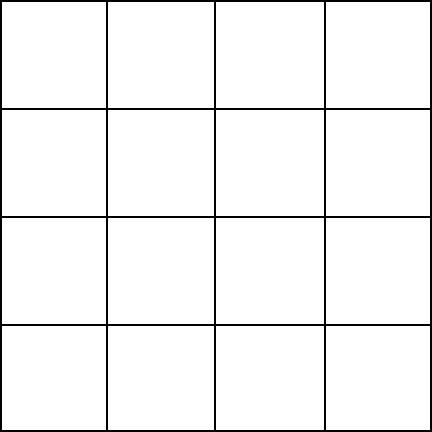## Counting Squares

How many squares of any size are in this grid?30.

There are:

16 1×1 squares
9 2×2 squares
4 3×3 squares
1 4×4 square (the whole shape)

Hence, 16 + 9 + 4 + 1 = 30.

Posted in Brain Teasers